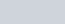Prove that the points A (4, 3),
Question:

Prove that the points A (4, 3), (6, 4), C (5, −6) and D (3, −7) in that order are the vertices of a parallelogram.

Solution:

Given points A (4, 3), B (6, 4), C (5, −6) and (3, −7).

We have to prove that these points form vertices of a parallelogram.

The above four points will form 4 line segments.

We know that length of a line segment having coordinates$=\sqrt{\left(x_{2}-x_{1}\right)^{2}+\left(y_{2}-y_{1}\right)^{2}}$

Therefore

$A B=\sqrt{(6-4)^{2}+(4-3)^{2}}$

$=\sqrt{4+1}$

$=\sqrt{5}$

$B C=\sqrt{(5-6)^{2}+(-6-4)^{2}}$

$=\sqrt{1+100}$

$=\sqrt{101}$

$C D=\sqrt{(3-5)^{2}+(-7-(-6))^{2}}$

$=\sqrt{4+1}$

$=\sqrt{5}$

$A D=\sqrt{(4-3)^{2}+(3-(-7))^{2}}$

$=\sqrt{1+100}$

$=\sqrt{101}$

It can be seen that AB = CD , BC = AD

Since opposite sides are equal, ABCD is a parallelogram

Hence proved.